Contents >> Applied Mathematics >> Numerical Methods >> Numerical Differentiaon >> Introduction

 Numerical differentiation - Introduction Introduction At solution of engineer-technical and other applied problems often happens it is necessary to find the certain order derivative from tabular given function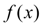. Besides sometimes by virtue of complexity of analytical expression of functionits direct differentiation is too inconvenient. In these cases numerical differentiation is usually used. Here there is set of various receptions and ways [1 – 3]. One of the simplest formulas for calculation of functionderivative is the formula of calculation of derivative through equidistant units [2, 3]: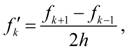(1) where h – step,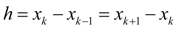,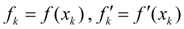. Sometimes given function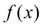on interesting us segment [ a , b ] is replaced with some interpolating function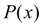, more often polynomial, and then is put :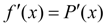,        (2) at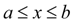. Similarly higher orders derivatives of functionare calculated. If an error of interpolating functionis known: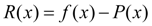,        (3) then error of derivative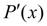is equal: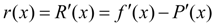,        (4) i.e. error of interpolating function derivative is equal to derivative of this function error . It is fair and for derivative of higher orders. Generally speaking, numerical differentiation is operation of less exact, than interpolation of function, differently affinity to each other ordinates of functionsandon segment [ a , b ] yet does not guarantee affinity on this segment of their derivatives, i.e. a small divergence of angular coefficients of tangents to considered curves at the same values of argument (Fig.1).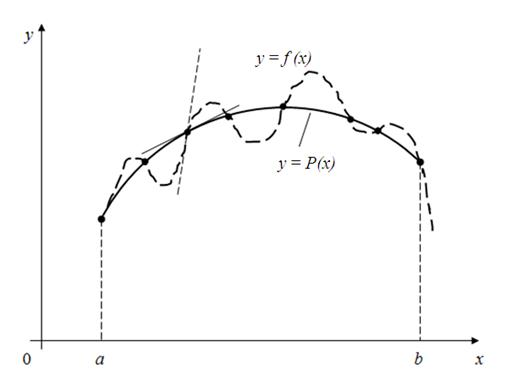Fig. 1. Difference in derivatives of given function y = f ( x ) and interpolating function y = P ( x )

 < Previous Contents Next >